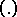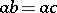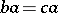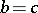# Groupoid

(diff) ← Older revision | Latest revision (diff) | Newer revision → (diff)
Jump to: navigation, search

A universal algebra with one binary operation. It is the broadest class of such algebras: groups, semi-groups, quasi-groups — all these are groupoids of a special type. An important concept in the theory of groupoids is that of isotopy of operations. On a setlet there be defined two binary operations, denoted byand; they are isotopic if there exist three one-to-one mappings,andofonto itself such thatfor all. A groupoid that is isotopic to a quasi-group is itself a quasi-group; a groupoid with a unit element that is isotopic to a group, is also isomorphic to this group. For this reason, in group theory the concept of isotopy is not used: For groups isotopy and isomorphism coincide.

A groupoid with cancellation is a groupoid in which either of the equations,implies, where,andare elements of the groupoid. Any groupoid with cancellation is imbeddable into a quasi-group. A homomorphic image of a quasi-group is a groupoid with division, that is, a groupoid in which the equationsandare solvable (but do not necessarily have unique solutions).

A set with one partial binary operation (i.e. one not defined for all pairs of elements) is said to be a partial groupoid. Any partial subgroupoid of a free partial groupoid is free.

How to Cite This Entry:
Groupoid. Encyclopedia of Mathematics. URL: http://encyclopediaofmath.org/index.php?title=Groupoid&oldid=17553
This article was adapted from an original article by V.D. Belousov (originator), which appeared in Encyclopedia of Mathematics - ISBN 1402006098. See original article